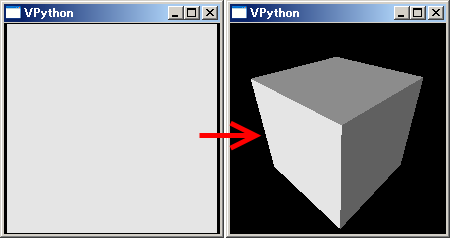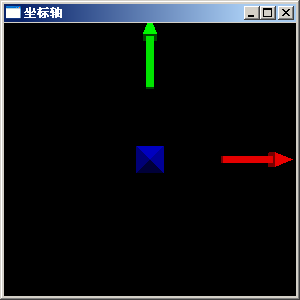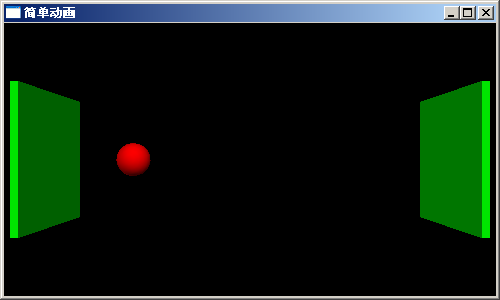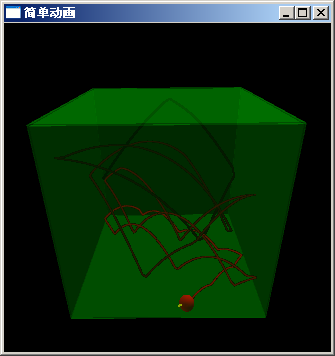# Visual-制作3D演示动画

Visual 是Python的一个简单易用的3D图形库，使用它可以快速创建3D场景、动画。和TVTK相比它更加适合于创建交互式的3D场景，而TVTK则更加适合于数据的3D图形化显示。在本节中将通过一个实例简单的介绍如何使用Visual制作3D动画。

## 场景、物体和照相机

```from visual import *
box()
```• 立方体的3D空间的坐标为 0, 0, 0，即坐标原点
• 立方体的大小为1, 1, 1
• 立方体的颜色为白色

```# -*- coding: utf-8 -*-
from visual import *
display(title=u"坐标轴".encode("gb2312"), width=300, height=300)
arrow(pos=(1,0,0), axis=(1,0,0), color=(1,0,0))
arrow(pos=(0,1,0), axis=(0,1,0), color=(0,1,0))
arrow(pos=(0,0,1), axis=(0,0,1), color=(0,0,1))
```VPython照相机的缺省位置，红绿蓝分别表示X,Y,Z轴

• 箭头的起点坐标用pos关键字参数指定，分别为(1,0,0), (0,1,0), (0,0,1)，坐标用3元组元表示。这三个坐标都在坐标轴上。
• 箭头的方向和长度使用axis关键字参数指定，其值为3D空间的矢量，矢量也是用三元组元表示，程序中所用的三个矢量正好是三个坐标轴的方向，长度为1。
• 通过color参数指定箭头物体的颜色，颜色也是用三元组元表示，取值范围为0到1，分别表示红、绿、蓝三色的成分。

• 窗口的中心为坐标原点
• x轴为从左到右
• y轴为从下到上
• z轴为从屏幕里到屏幕外

## 简单动画

 ``` 1 2 3 4 5 6 7 8 9 10 11 12 13 14 15 16 17``` ```# -*- coding: utf-8 -*- from visual import * display(title=u"简单动画".encode("gb2312"), width=500, height=300) ball = sphere(pos=(-5,0,0), radius=0.5, color=color.red) wall_right = box(pos=(6,0,0), size=(0.1, 4, 4), color=color.green) wall_left = box(pos=(-6,0,0), size=(0.1, 4, 4), color=color.green) dt = 0.05 ball.velocity = vector(6, 0, 0) while True: rate(1/dt) ball.pos = ball.pos + ball.velocity*dt if ball.x > wall_right.x-ball.radius or ball.x < wall_left.x+ball.radius: ball.velocity.x *= -1 ```## 盒子中反弹的球

 ``` 1 2 3 4 5 6 7 8 9 10 11 12 13 14 15 16 17 18 19 20 21 22 23 24 25 26 27 28 29 30 31 32 33 34 35 36 37 38 39 40 41 42 43 44 45 46 47 48 49 50 51 52 53 54 55 56 57 58 59 60 61 62 63 64``` ```# -*- coding: utf-8 -*- from visual import * display(title=u"简单动画".encode("gb2312"), width=500, height=500) # 创建球体和6个墙面，墙面设置为半透明，以观察球体的运动轨迹 ball = sphere(pos=(-5,0,0), radius=0.5, color=color.red) wall_right = box(pos=(6,0,0), size=(0.1, 12, 12), color=color.green, opacity = 0.2) wall_left = box(pos=(-6,0,0), size=(0.1, 12, 12), color=color.green, opacity = 0.2) wall_front = box(pos=(0,-6,0), size=(12, 0.1, 12), color=color.green, opacity = 0.2) wall_back = box(pos=(0,6,0), size=(12, 0.1, 12), color=color.green, opacity = 0.2) wall_bottom = box(pos=(0,0,-6), size=(12, 12, 0.1), color=color.green, opacity = 0.2) wall_top = box(pos=(0,0,6), size=(12, 12, 0.1), color=color.green, opacity = 0.2) dt = 0.05 g = 9.8 # 重力加速度 f = 0.9 # 反弹能量保持系数，1.0表示完全反弹 ball.velocity = vector(8, 6, 12) bv = arrow(pos = ball.pos, axis=ball.velocity*0.2, color=color.yellow) ball.trail = curve(color=ball.color) trail_color = 0 # 轨迹的颜色 while True: rate(1/dt) # 重力加速度改变z轴方向的速度，不存在反弹时修改速度 ball.velocity.z -= g * dt # 根据速度修改球体的位置 ball.pos += ball.velocity * dt ## 速度为正时判断正方向的墙，速度为负时判断负方向的墙 ## 处理反弹时需要修正球的位置，使它正好和墙面接触 # 处理左右墙的反弹 if ball.velocity.x > 0 and ball.x >= wall_right.x - ball.radius: ball.x = wall_right.x - ball.radius ball.velocity.x *= -f if ball.velocity.x < 0 and ball.x <= wall_left.x + ball.radius: ball.x = wall_left.x + ball.radius ball.velocity.x *= -f # 处理前后墙的反弹 if ball.velocity.y > 0 and ball.y >= wall_back.y - ball.radius: ball.y = wall_back.y - ball.radius ball.velocity.y *= -f if ball.velocity.y < 0 and ball.y <= wall_front.y + ball.radius: ball.y = wall_front.y + ball.radius ball.velocity.y *= -f # 处理上下墙的反弹 if ball.velocity.z > 0 and ball.z >= wall_top.z - ball.radius: ball.z = wall_top.z - ball.radius ball.velocity.z *= -f elif ball.velocity.z < 0 and ball.z <= wall_bottom.z + ball.radius: ball.z = wall_bottom.z + ball.radius ball.velocity.z *= -f # 更新速度箭头的位置和方向 bv.pos = ball.pos bv.axis = ball.velocity*0.2 # 添加球的轨迹点 ball.trail.append( pos = ball.pos, color = (trail_color, 0, 0)) trail_color += 1.0/30.0*dt # 30秒后颜色变为全红 if trail_color > 1.0: trail_color = 1.0 ``````bv = arrow(pos = ball.pos, axis=ball.velocity*0.1, color=color.yellow)
```

```ball.trail = curve(color=ball.color)
```

```if ball.velocity.x > 0 and ball.x >= wall_right.x - ball.radius: